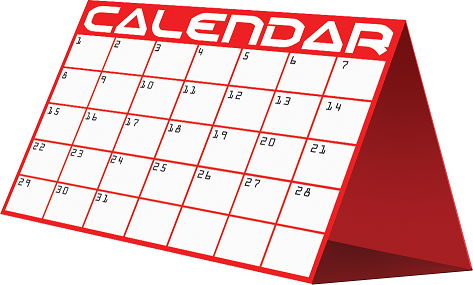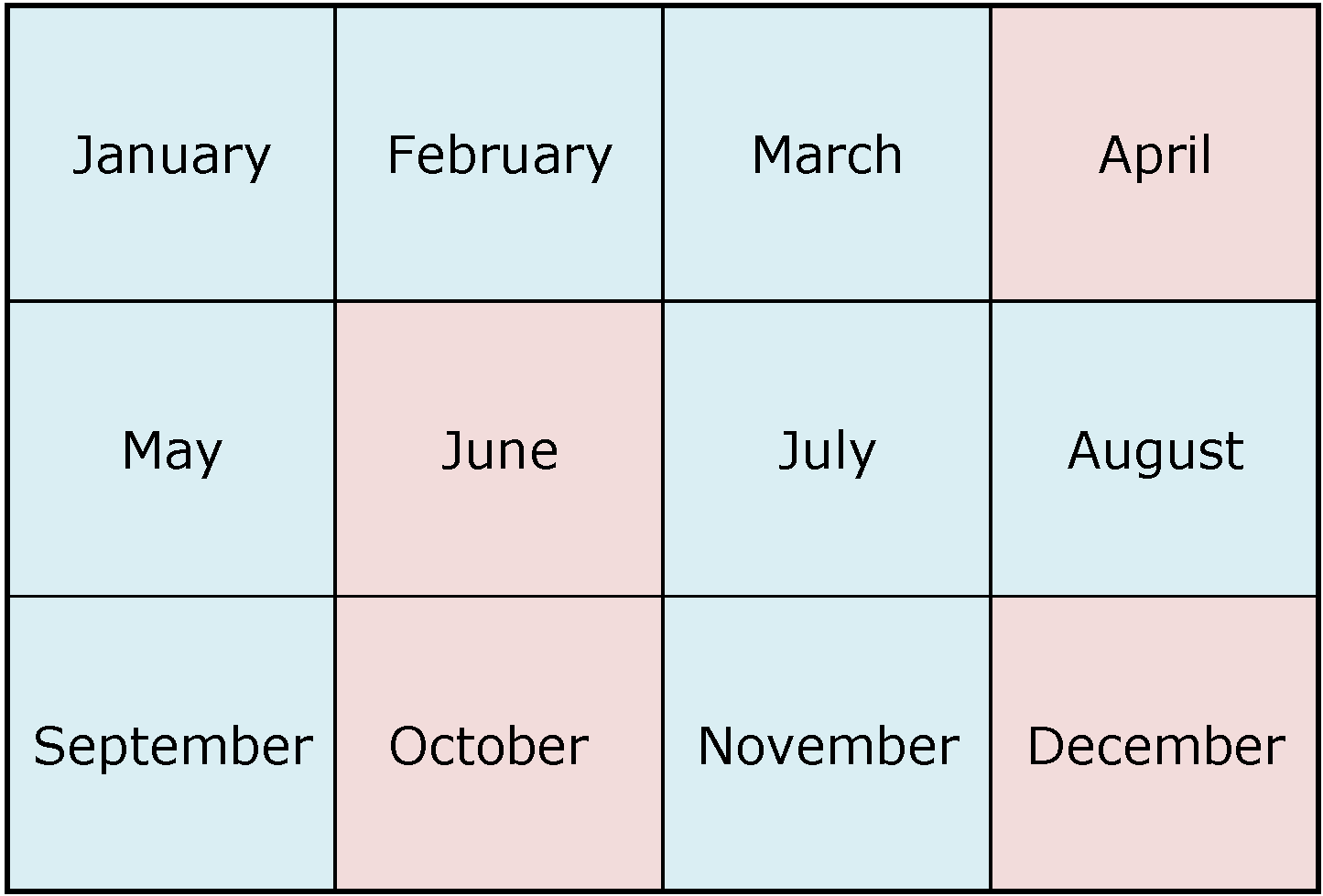ISSN 2284-7995, ISSN Online 2285-3952

## HERITABILITY OF TRAITS OF THE TYPE LINEAR ASSESSMENT AND THEIR GENETIC ASSOCIATION WITH COW'S MILK YIELD OF UKRAINIAN DAIRY BREEDS

###### Published in Scientific Papers. Series "Management, Economic Engineering in Agriculture and rural development", Vol. 20 ISSUE 1 Written by Leontii KHMELNYCHYI, Victoria VECHORKA, Anatolyi SALOHUB, Serhii KHMELNYCHYI, Igor RUBTSOV

The heritability of linear type traits and phenotypic correlations between them and milk yield of cows of Ukrainian Red-and-White (URW) and Black-and-White (UBW) dairy breeds was evaluated. Cows firstborn were estimated on the 100-point scale by such group traits: dairy type, body, limbs, udder and final score. 18 individual descriptive traits have been taken into account on a 9-point scale. By reliable significance confirmed sufficient degree of genetic determination group and most descriptive linear traits of URW cows (h2 = 0.288-0.426 and h2 = 0.1610.422) and UBW (h2 = 0.262-0.453 and h2 = 0.128-0.434) testifying about feasibility and efficiency of mass selection dairy cattle by conformation. A reliable level (P <0.001) of positive correlation was determined between traits of dairy type URW and UBW (r = 0.412 and 0.468), body (r = 0.433 and 0.487) and udder (r = 0.453 and 0.474) and milk yield for 305 days of lactation. A reliable positive correlation was found between the final score and the yield value (r = 0.465 and 0.494). Lower correlation was found between limb condition and milk yield in URW and UBW cows, respectively (r = 0.122; P <0.01 and r = 0.205; P <0.001). Positive genetic association with milk yield was determined by the following descriptive traits of the type in URW and UBW, respectively: height (r = 0.312 and 0.278), body depth (r = 0.288 and 0.404), angularity (r = 0.504 and 0.486), rump width ( r = 0.252 and 0.374), pelvic limb posture (r = 0.354 and 0.384), front udder attachment (r = 0.466 and 0.455), rear udder attachment height (r = 0.325 and 0.373), central ligament (r = 0.278 and 0.267) and locomotion (r = 0.286 and 0.275). A negative and reliable correlation was found between the trait of fatness (BCS) and yield, which was -0.366 and -0.389, respectively (P <0.001). Reliable correlation variability of group and most descriptive conformation traits with milk yield for the first lactation confirmed the possibility of unilateral breeding of dairy cattle by type.### Find ArticleNext Issue will be published in AprilFour issues are published every year.

The publisher is not responsible for the opinions published in the Volume. They represent the authors’ point of view.

© 2019 To be cited: Scientific Papers. Series “Management, Economic Engineering in Agriculture and Rural Development“.